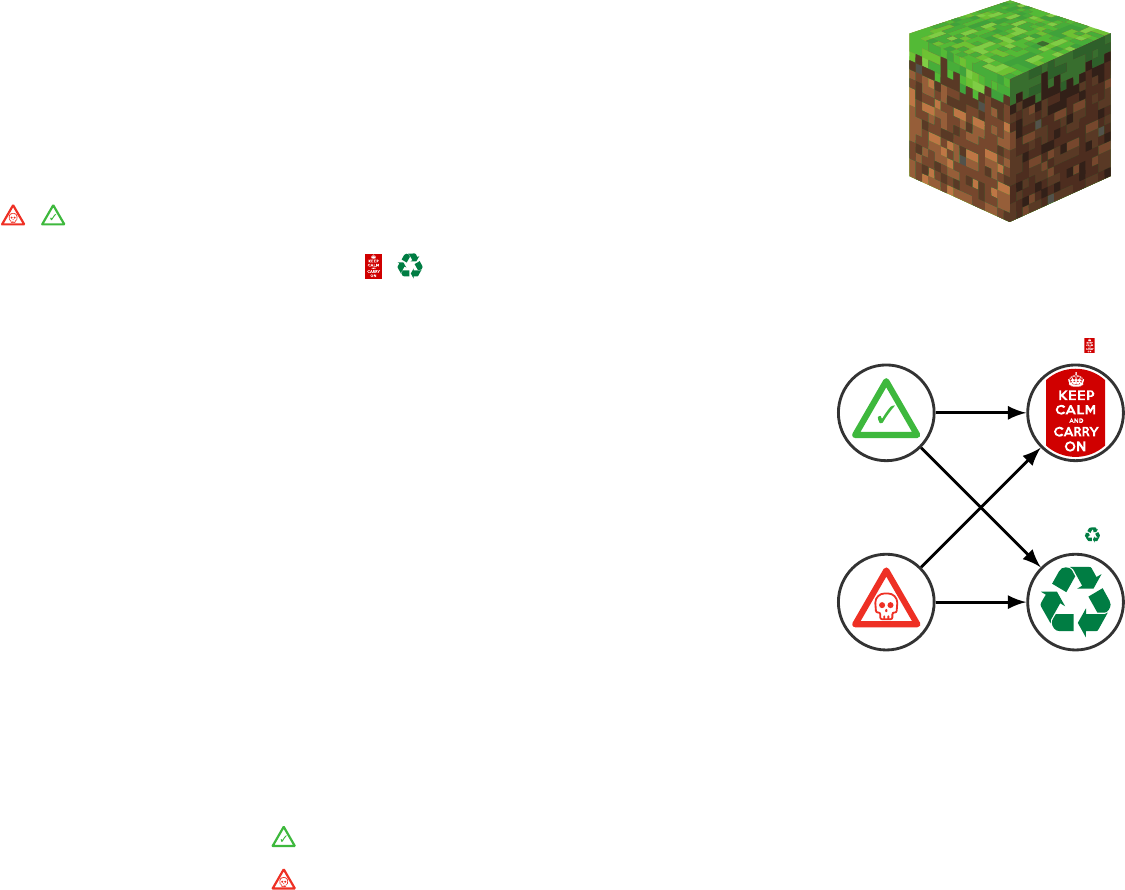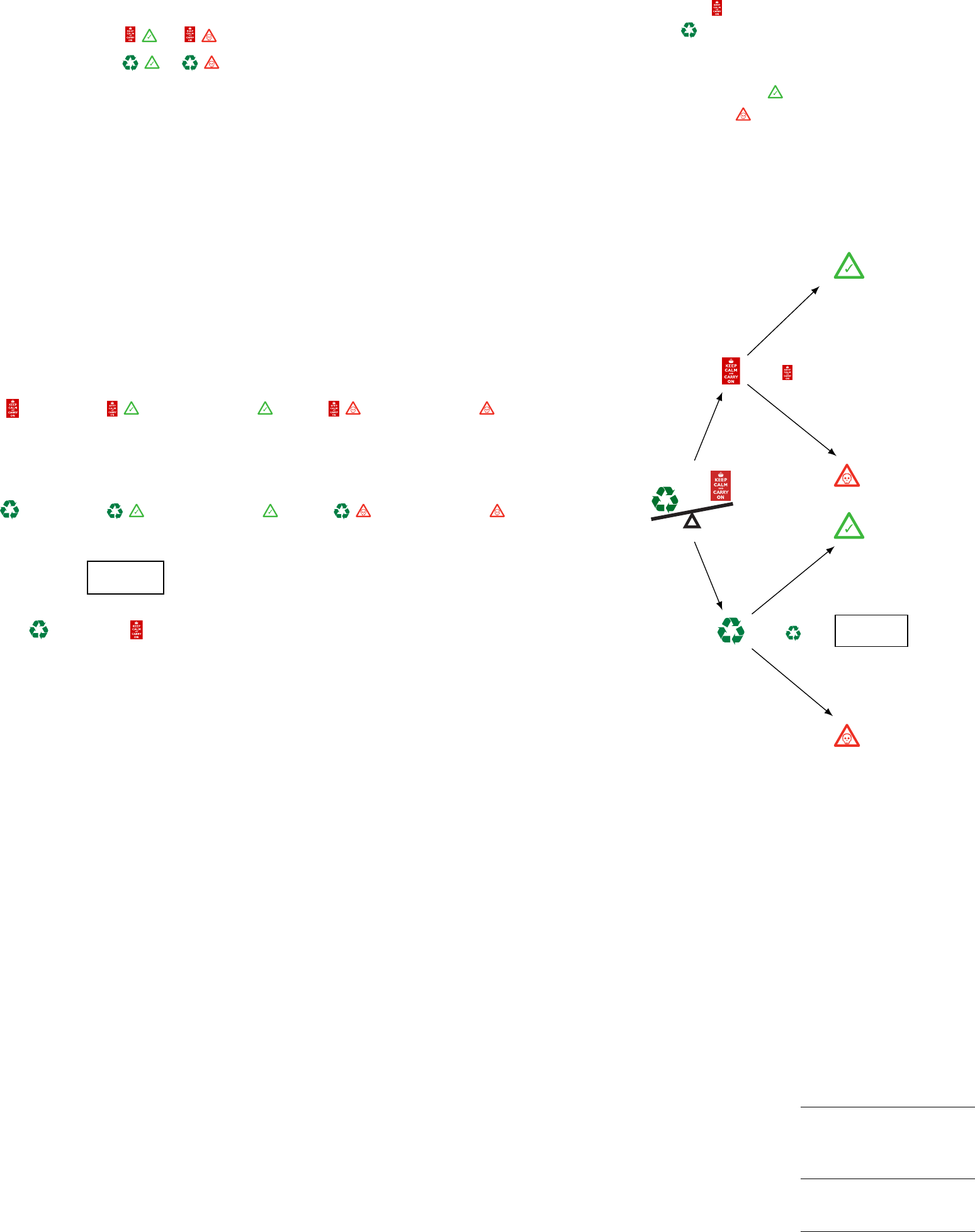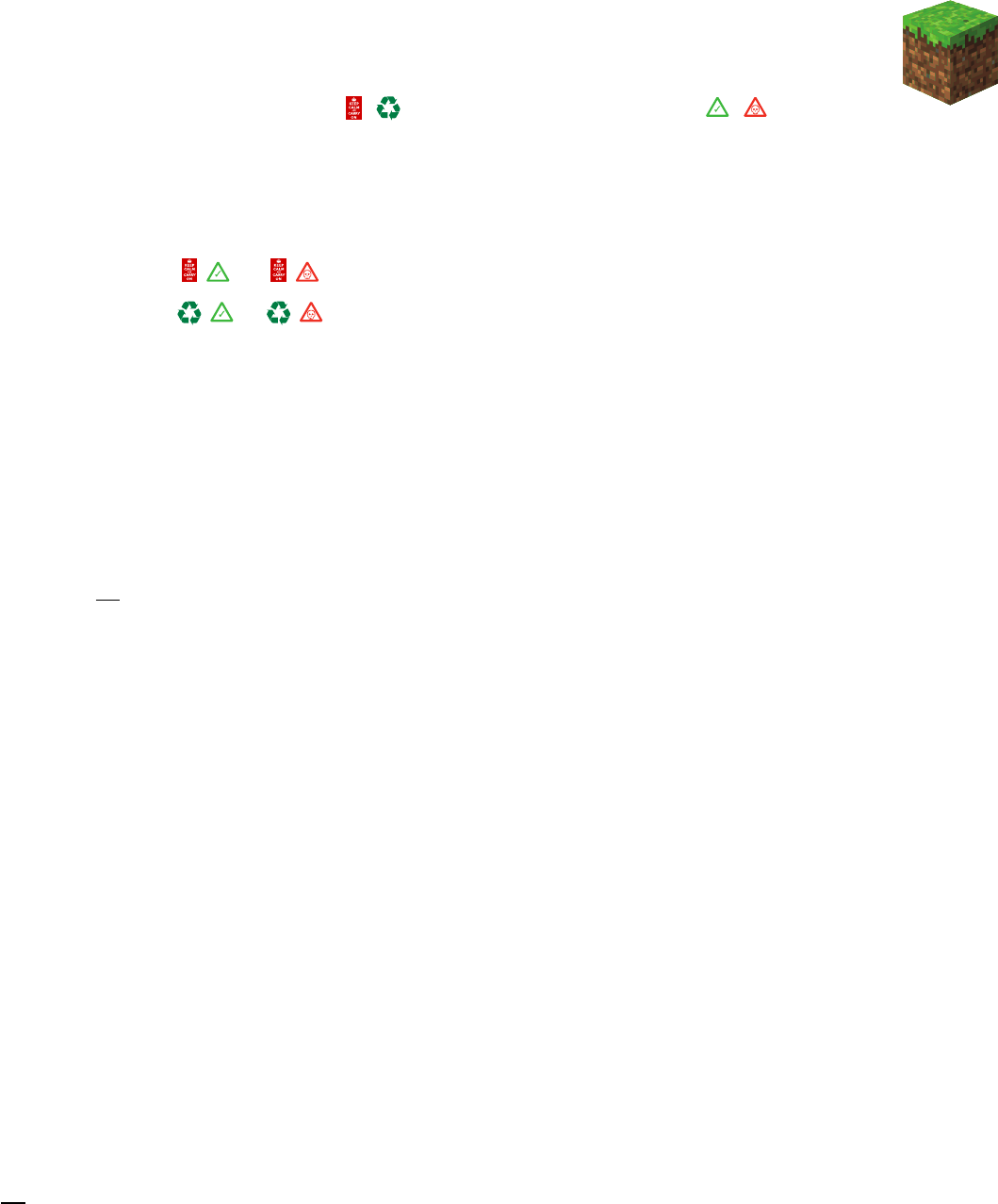14
Decisions in Uncertain Contexts
This chapter explores how to m ake rational deci si ons regar di n g
actions to be t aken in a context where the state variables on which
a dec i si on is based are themselves uncer t ain . Basic contextual
notions will be presented through an illustrative example before
introducing the formal mathematical notation associated with
rational decisions and value of information.
14.1 Introductory Example
(a) Given an
m
3
of possibly
contaminated soil.
Pr = 0.9
Pr = 0.1
\$0
9\$100
9\$10K
9\$100
E[\$| ]=9\$1K
E[\$| ]=9\$100
(b) State probabilities, values, and
expected values.
Figure 14.1: Decision context for a soil
contamination example.
In or de r to introdu ce the noti ons associat ed wit h utility theory,
we re vi s it the soil contamination example presented in
§
8.2.5,
where we have a cubic meter of potentially contaminated soil . The
two possi b l e states
x 2{, }
are e i t he r contaminated or not
contaminated, and the two possi bl e acti ons
a 2{, }
are e i t he r to
do nothing or t o send the soil to a recycling plant where it is going
to be decontaminated. A soi l sampl e is deﬁned as contaminated
if the pollutant concentration
c
exceeds a threshold value
c>
. Th e issu e is that for most m
3
in an indu st r i al sit e, the re
are n o observations made in order to verify whether or not it is
contaminated. Here, we rely on a regre ssi on model buil t from a set
of d is cr et e obser vations to predi ct for any
x, y, z
coordinates what
our knowledge of t he contaminant conc entration is as descri bed
by it s expected value and variance. In this example, our current
knowledge indicates that, for a speciﬁc m
3
,
Pr(C
) Pr(X = )=0.9
Pr(C>
) Pr(X = )=0.1.
The optimal decision regarding the action to choose depends
on t he value incurr ed by taking an action given the state
x
.In
this example we have two states and two actions, so there are four
possibilities to be deﬁned, as illustrate d in ﬁgure 14.1. The incurredj.-a. goulet 230
value (\$) for each p ai r of st at e s x and actions taken a are
\$(a, x)=\$
, ,
, ,
\$0 \$10K
\$100 \$100
,
where doing nothing when the soil is n ot contaminated incurs no
cost, decontaminating incurs a cost of
\$
100/m
3
whether or not t h e
soil is contaminated, and omitting the decontamination when it is
actually contaminated (i.e.,
c>
) i n cu rs a cost of
\$
10 K in
future legal fees and compensatory damages.
Actions
Do nothing
Recycle
States
Not contaminated
Contaminated
:\$100
Pr=0.1
:\$100
Pr=0.9
:\$10K
Pr=0.1
:\$0
Pr=0.9
E[\$| ]= \$100
E[\$| ]=
\$1 000
Figure 14.2: Decision tree for the soil
contamination example.
Figure 14.2 depicts a decision tree illustrating the actions along
with the probability of each out c ome. The opti mal acti on to be
taken must be sele ct e d based on the expect ed value condit i onal on
each action,
E[\$| ] = \$( , ) Pr(X = ) + \$( , ) Pr(X = )
= (\$0 0.9) + (\$10K 0.1)
= \$1 000
E[\$| ] = \$( , ) Pr(X = ) + \$( , ) Pr(X = )
=(\$100 0.9) + (\$100 0.1)
= \$100 .
Because
E
[\$
|
]
> E
[\$
|
], the optimal action is thus t o send the
soil to a recycl i ng plant where it will be decontaminated. As you
can e x pect , changing the probabi l i ty of each state or changing the
incurred relative value (
\$
) f or a pair of states
x
and actions taken
a
will inﬂuence the optimal action to be taken. The next section
presents the utility theory, which formalizes the method employed
in this introductory ex amp le .
14.2 Utility Theory
Utility theory deﬁnes how rational decisions are made. This section
presents the nomenclature associated with utility theory, it formal-
izes what a ration al deci si on is, and it presents its fund amental
axioms.
14.2.1 Nomenclature
A = {a
1
, ··· , a
A
}
Asetofpossible
actions
x 2XZ or R
An outcome from
asetofpossible
states
Pr(X = x) Pr(x)
Probability of a
state x
U(a, x)
Utility given a
state
x
and an
action a
A decision consists in the task of cho os i ng an action a
i
from a set of
possible actions
A
=
{
a
1
,
a
2
, ··· ,
a
A
}
. Th i s decis i on is based on our
knowledge of on e or several state variables
x
, wh i ch can either be
discrete or continuous. Our knowledge of a s t ate variable is eithe r
described by its probability density
X f
(
x
) or mass functi on
X p
(
x
)=
Pr
(
X
=
x
). The utility
U
(
a, x
) q u antiﬁes th e relat i veprobabilistic machine learning for civil engineers 231
preferences we have f or the joint result of taking an action
a
while
being in a state x.
Soil contamination ex am pl e For the example presented in the in-
troduction of this chapter, the actions and s tat e s can be formulated
as bi n ary disc rete variables;
a 2{, }{
0
,
1
}
and
x 2{ , }
{
0
,
1
}
. Th e probabi li ty of each state is
Pr
(
X
=
x
)=
{
0
.
9
,
0
.
1
}
, an d
the utility of each pair of act i ons , and states, is
U(a, x)=U
, ,
, ,
\$0 \$10K
\$100 \$100
.
14.2.2 Rational Decisions
In t h e context of the utility theory, a rational decision is deﬁned
as a decisi on that maximi z es the expected utility. In an uncertain
context, the perceived beneﬁt of an action a
i
is measured by t he
expected ut ility,
U
(
a
)
E
[
U
(
a, X
)]. When
X
is a disc re t e random
variable so that x 2X= {1, 2, ··· , X},theexpectedutilityis
E[U(a, X)] =
X
x2X
U(a, x) ·p(x).
X
is a continuous rand om variable, the expect e d
utility is
E[U(a, X)] =
Z
U(a, x) ·f(x)dx.
The opti m al action
a
is the on e that maxim i z es the expected
utility,
a
= arg max
a2A
E[U(a, X)],
U(a
) E[U(a
,X)] = max
a2A
E[U(a, X)].
14.2.3 Axioms of Utility Theory
The axioms of uti l ity the or y are based on the concepts of lotteries.
A lot t er y
L
is deﬁned by a set of possible outcomes
x 2X
=
{
1
,
2
, ··· , X}
, each having its own probability of occurre nc e
p
X
(
x
)=
Pr(X = x), so that
L =[{p
X
(1),x=1}; {p
X
(2),x=2}; ··· ; {p
X
(X),x=X}]. (14.1)
A dec i si on is a choice made between several lott er ie s. For the soil
contamination example, there are two lotteries, each one corre-
sponding to a possi bl e acti on. If we choose to send the soil to a
recycling facility, we are cert ain of the outcome, that is, the soil is